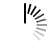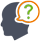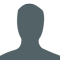Diwali Bonanza | Get 30-Days Free Fun-Filled Learning | Subscribe Now1800-833-6464 Buy Activation Why Tutorix Features Login

#DoubtTushar

In figure below, $∠\ ABC\ =\ 90^o$ and $BD\ ⊥\ AC$. If $BD\ =\ 8\ cm$, and $AD\ =\ 4\ cm$, find $CD$.Solutions :Akhilesh

Given:

In the given figure $∠ \ ABC\ =\ 90^o$ and $BD\ âŠ¥\ AC$.

$BD\ =\ 8\ cm$, and $AD\ =\ 4\ cm$.

To do:

We have to find $CD$.

Solution:

In $\vartriangle ABD$ and $\vartriangle BCD$,

$\angle ABD+\angle DBC=90^o$

$\angle C+\angle DBC=90^o$

This implies,

$\angle ABD=\angle C$

In $\vartriangle ABD$ and $\vartriangle BCD$,

$\angle ABD=\angle C$

$\angle ADB=\angle BDC=90^o$

Therefore,

$\vartriangle DBA∠¼\vartriangle DCB$  (By AA similarity)

$\frac{BD}{CD} = \frac{AD}{BD}$   (Corresponding parts of similar triangles are proportional)

$BD^2 = AD \times DC$

$(8)^2 = 4 \times DC$

$DC = \frac{64}{4} = 16\ cm$

The measure of $CD$ is $16\ cm$.

0

﻿